Home    |    Teacher    |    Parents    |    Glossary    |    About UsEmail this page to a friendResources· Cool Tools · Formulas & Tables · References · Test Preparation · Study Tips · Wonders of MathSearchGamma Function/b> (Math | Calculus | Integrals | Special Functions | Gamma Function)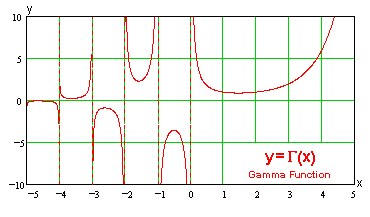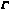(x) =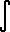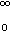e -t t(x-1) dt(x) = r xe -rt t (x-1) dt(x) = 2e (-t^2) t (2x-1) dt ((x)(y) ) / ((x) +(y) ) = Beta(x,y)(x+1) = x(x)(x+1) = x!(0+) = +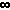(1/2) =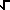(PI)(z)(1-z) = PI csc(PI z)'(1) = - gamma (Euler's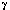constant = 0.577215...)'(x) =(x) lim (n-->) [ ln(n) -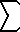(k=0..n-1) 1/(x+k) ]Function Expansions(x+1) = lim (k-->) kx 1*2*3*..*k (x+1)(x+2)*..*(x+k)
Stirling's Asymptotic Series(x+1) =(2PIx) xx e -x { 1 + 1/(12x) + 1/(288 x2) - 139/(51840 x3) - 571/(2488320 x4) + 163879/(209018880 x5) + 5246819/(75246796800 x6) - 534703531/(902961561600 x7) - ...}
(this is only an approximation, see note.)Incomplete Gamma Functions:(x, a) =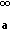e -t t (x-1) dt(x, a) =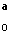e -t t (x-1) dt(x, a) +(x, a) =(x)